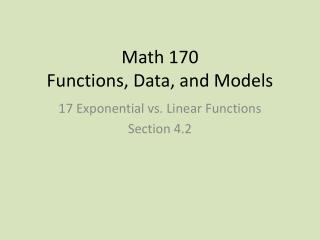DownloadDownload PresentationMath 170 Functions, Data, and Models

# Math 170 Functions, Data, and Models

Download Presentation## Math 170 Functions, Data, and Models

- - - - - - - - - - - - - - - - - - - - - - - - - - - E N D - - - - - - - - - - - - - - - - - - - - - - - - - - -
##### Presentation Transcript

1. Math 170 Functions, Data, and Models 17 Exponential vs. Linear Functions Section 4.2

2. Basic Concepts • A function is linear if it changes at a constant absolute rate. • is the initial value; is the rate of change • A function is exponential if it changes at a constant percentage rate. • is the initial value; is the growth factor; is the growth rate

3. Table Example • For each function and each adjacent input interval, what is the change? • Find a formula for the constant rate of change function. • For each function and each adjacent input interval, what is the percent change? • Find a formula for the constant relative rate of change function. • Quickly sketch graphs of the two functions. • Find the (maximum) domain and range of the two functions.

4. Graph Examples • Find an equation for the green graph. • Find an equation for the red graph.

5. Population vs. Food (4.2 Example 3) • The population of a country is initially 2 million people and is increasing at 4% per year. The country's annual food supply is initially adequate for 4 million people and is increasing at a constant rate adequate for an additional 0.5 million people per year. • Based on these assumptions, in approximately what year will this country first experience shortages of food? • If the country doubled its initial food supply, would shortages still occur? If so, when? (Assume the other conditions do not change). • If the country doubled the rate at which its food supply increases, in addition to doubling its initial food supply, would shortages still occur? If so, when? (Again, assume the other conditions do not change.)

6. Olympic Times (4.2 Problem #37) • Short track speed skating became a Winter Olympic event in 1994, and ChaeJi‐Hoon of Korea won the event that year with a time of seconds. In 2006, ApoloOhno of the US won the event with a time of seconds. Find a formula for the predicted winning time in the speed skating event as a function of the number of years since 1994, and predict the winning time in 2018, if we assume the decrease in time is (a) linear, and (b) exponential.